# fairnessThresholder

Optimize classification threshold to include fairness

Since R2023a

## Description

`fairnessThresholder` searches for an optimal score threshold to maximize accuracy while satisfying fairness bounds. For observations in the critical region below the optimal threshold, the function adjusts the labels so that the fairness constraints hold for the reference and nonreference groups in the sensitive attribute. After you create a `fairnessThresholder` object, you can use the `predict` and `loss` object functions on new data to predict fairness labels and calculate the classification loss, respectively.

## Creation

### Syntax

``fairnessMdl = fairnessThresholder(Mdl,Tbl,AttributeName,ResponseVarName)``
``fairnessMdl = fairnessThresholder(Mdl,X,attribute,Y)``
``fairnessMdl = fairnessThresholder(Mdl,attribute,threshold)``
``fairnessMdl = fairnessThresholder(___,Name=Value)``

### Description

example

````fairnessMdl = fairnessThresholder(Mdl,Tbl,AttributeName,ResponseVarName)` optimizes the score threshold for a binary classifier `Mdl` while satisfying fairness bounds. The function tries a vector of thresholds for classifying observations in the validation data table `Tbl` with the class labels in the `ResponseVarName` table variable. For observations in the critical region below the optimal threshold, the function adjusts the labels so that the fairness constraints hold for the reference and nonreference groups in the `AttributeName` sensitive attribute. For more information, see Reject Option-Based Classification.```

example

````fairnessMdl = fairnessThresholder(Mdl,X,attribute,Y)` uses the numeric predictor data `X`, the sensitive attribute specified by `attribute`, and the binary response specified by `Y`.```
````fairnessMdl = fairnessThresholder(Mdl,attribute,threshold)` uses the specified `threshold` as the score threshold instead of solving an optimization problem. Select a fixed threshold based on domain expertise.```

example

````fairnessMdl = fairnessThresholder(___,Name=Value)` specifies options using one or more name-value arguments in addition to any of the input argument combinations in previous syntaxes. For example, specify the bias metric by using the `BiasMetric` name-value argument.```

### Input Arguments

expand all

Binary classifier, specified as a full or compact classification model object or a function handle.

Validation data set, specified as a table. Each row of `Tbl` corresponds to one observation, and each column corresponds to one variable. The table must include all predictor variables used to train `Mdl`, the sensitive attribute, and the response variable. The table can include additional variables, such as observation weights. Multicolumn variables and cell arrays other than cell arrays of character vectors are not allowed.

Data Types: `table`

Sensitive attribute name, specified as the name of a variable in `Tbl`. You must specify `AttributeName` as a character vector or a string scalar. For example, if the sensitive attribute is stored as `Tbl.Attribute`, then specify it as `"Attribute"`.

The sensitive attribute must be a numeric vector, logical vector, character array, string array, cell array of character vectors, or categorical vector.

Data Types: `char` | `string`

Response variable name, specified as the name of a variable in `Tbl`. You must specify `ResponseVarName` as a character vector or a string scalar. For example, if the response variable is stored as `Tbl.Y`, then specify it as `"Y"`.

The response variable must be a numeric vector, logical vector, character array, string array, cell array of character vectors, or categorical vector. The data type must be the same as the data type of the response variable used to train `Mdl`.

Data Types: `char` | `string`

Validation predictor data, specified as a numeric matrix. Each row of `X` corresponds to one observation, and each column corresponds to one predictor variable.

• `X`, `attribute`, and `Y` must have the same number of rows.

• The columns of `X` must have the same order as the predictor variables used to train `Mdl`.

Data Types: `single` | `double`

Sensitive attribute, specified as a numeric column vector, logical column vector, character array, string array, cell array of character vectors, or categorical column vector.

• `X`, `attribute`, and `Y` must have the same number of rows.

• If `attribute` is a character array, then each row of the array must correspond to a group in the sensitive attribute.

Data Types: `single` | `double` | `logical` | `char` | `string` | `cell` | `categorical`

Class labels, specified as a numeric column vector, logical column vector, character array, string array, cell array of character vectors, or categorical column vector.

• `X`, `attribute`, and `Y` must have the same number of rows.

• If `Y` is a character array, then each row of the array must correspond to a class label.

• The data type of `Y` must be the same as the data type of the response variable used to train `Mdl`.

• If `Mdl` is a classification model object, then the distinct classes in `Y` must be a subset of the classes in `Mdl.ClassNames`.

Data Types: `single` | `double` | `logical` | `char` | `string` | `cell` | `categorical`

Score threshold, specified as a numeric scalar. `fairnessThresholder` adjusts the label for each observation whose maximum score is less than the `threshold` value.

• If `Mdl` or its `predict` object function returns classification scores that are posterior probabilities, then specify a `threshold` value in the range [0.5, 1].

• If the `predict` object function of `Mdl` returns classification scores in the range (–∞,∞), then specify a nonnegative `threshold` value.

Data Types: `single` | `double`

Name-Value Arguments

Specify optional pairs of arguments as `Name1=Value1,...,NameN=ValueN`, where `Name` is the argument name and `Value` is the corresponding value. Name-value arguments must appear after other arguments, but the order of the pairs does not matter.

Example: ```fairnessThresholder(Mdl,Tbl,"Gender","Smoker",BiasMetric="spd",BiasMetricRange=[–0.1 0.1])``` specifies to find a score threshold so that the statistical parity difference for the nonreference group in the `Gender` sensitive attribute is in the range [–0.1, 0.1].

Bias metric to use as a fairness constraint during the threshold optimization, specified as one of the metric names in this table.

Metric NameDescription
`"DisparateImpact"` or `"di"` (default)Disparate impact (DI)
`"AverageAbsoluteOddsDifference"` or `"aaod"`Average absolute odds difference (AAOD)
`"EqualOpportunityDifference"` or `"eod"`Equal opportunity difference (EOD)
`"StatisticalParityDifference"` or `"spd"`Statistical parity difference (SPD)

The `fairnessThresholder` function computes the bias metric for the nonreference group (that is, the complement of `ReferenceGroups`) and checks whether the value is within the bias metric bounds (`BiasMetricRange`).

Example: `BiasMetric="spd"`

Example: `BiasMetric="EqualOpportunityDifference"`

Data Types: `char` | `string`

Bounds on the bias metric to use as constraints during the threshold optimization, specified as a two-element numeric vector. This table describes the supported bias metric values and the default bias metric bounds for each bias metric.

Metric NameSupported Bias Metric ValuesDefault `BiasMetricRange` Value
`"DisparateImpact"` or `"di"`[0, ∞)[0.8, 1.25]
`"AverageAbsoluteOddsDifference"` or `"aaod"`[0, 1][0, 0.05]
`"EqualOpportunityDifference"` or `"eod"`[–1, 1][–0.05, 0.05]
`"StatisticalParityDifference"` or `"spd"`[–1, 1][–0.05, 0.05]

The `fairnessThresholder` function computes the bias metric (`BiasMetric`) for the nonreference group (that is, the complement of `ReferenceGroups`) and checks whether the value is within the bias metric bounds.

Example: `BiasMetricRange=[-0.1 0.1]`

Data Types: `single` | `double`

Groups in the sensitive attribute to use as the reference group when computing bias metrics, specified as a scalar or a vector. By default, `fairnessThresholder` chooses the most frequently occurring group in the validation data as the reference group. Each element in the `ReferenceGroups` value must have the same data type as the sensitive attribute.

The function uses a technique designed for binary sensitive attributes that contain a reference group and a nonreference group. Sensitive attribute groups not in the `ReferenceGroups` value form the nonreference group.

Example: `ReferenceGroups=categorical(["Husband","Unmarried"])`

Data Types: `single` | `double` | `logical` | `char` | `string` | `cell` | `categorical`

Label of the positive class, specified as a numeric scalar, logical scalar, character vector, string scalar, cell array containing one character vector, or categorical scalar. `PositiveClass` must have the same data type as the true class label variable.

The default `PositiveClass` value is the second class of the binary labels, according to the order returned by the `unique` function with the `"sorted"` option specified for the true class label variable.

Example: `PositiveClass=categorical(">50K")`

Data Types: `single` | `double` | `logical` | `char` | `string` | `cell` | `categorical`

Loss to minimize during the threshold optimization, specified as `"classiferror"`, `"classifcost"`, or a function handle.

This table lists the available loss functions. Specify one using its corresponding character vector or string scalar.

ValueDescriptionEquation
`"classifcost"`Observed misclassification cost
`$L=\frac{\sum _{j=1}^{n}C\left({k}_{j},{\stackrel{^}{k}}_{j}\right)\cdot I\left({y}_{j}\ne {\stackrel{^}{y}}_{j}\right)}{n}$`
`"classiferror"`Misclassified rate in decimal
`$L=\frac{\sum _{j=1}^{n}I\left({y}_{j}\ne {\stackrel{^}{y}}_{j}\right)}{n}$`
• C is the misclassification cost matrix, and I is the indicator function. If `Mdl` is a classification model object, the misclassification cost matrix corresponds to the `Cost` property of `Mdl`. If `Mdl` is a function handle, C is the default cost matrix, and the loss values for `"classifcost"` and `"classiferror"` are identical.

• yj is the true class label for observation j, and yj belongs to class kj.

• ${\stackrel{^}{y}}_{j}$ is the class label with the maximal predicted score for observation j, and ${\stackrel{^}{y}}_{j}$ belongs to class ${\stackrel{^}{k}}_{j}$.

• n is the number of observations in the validation data set.

To specify a custom loss function, you must specify `Mdl` as a classification model object. Use function handle notation (`@lossfun`), where the function has this form:

``lossvalue = lossfun(Class,Score,Cost)``

• The output argument `lossvalue` is a scalar.

• You specify the function name (`lossfun`).

• `Class` is an `n`-by-`K` logical matrix with rows indicating the class to which the corresponding observation belongs. `n` is the number of observations in `Tbl` or `X`, and `K` is the number of distinct classes in the response variable. The column order corresponds to the class order in `Mdl.ClassNames`. Create `Class` by setting `Class(p,q) = 1`, if observation `p` is in class `q`, for each row. Set all other elements of row `p` to `0`.

• `Score` is an `n`-by-`K` numeric matrix of classification scores. The column order corresponds to the class order in `Mdl.ClassNames`. `Score` is a matrix of classification scores, similar to the output of `predict`.

• `Cost` is a `K`-by-`K` numeric matrix of misclassification costs. For example, ```Cost = ones(K) – eye(K)``` specifies a cost of `0` for correct classification and `1` for misclassification.

Example: `LossFun="classifcost"`

Data Types: `char` | `string` | `function_handle`

Maximum number of threshold values to evaluate during the threshold optimization, specified as a positive integer. `fairnessThresholder` uses a vector of `min(n,MaxNumThresholds)` threshold values as part of the optimization process, where `n` is the number of observations in the validation data.

Example: `MaxNumThresholds=250`

Data Types: `single` | `double`

## Properties

expand all

Binary classifier, returned as a full or compact classification model object or a function handle.

Sensitive attribute, returned as a variable name, numeric column vector, logical column vector, character array, cell array of character vectors, or categorical column vector.

• If you use a table to create the `fairnessThresholder` object, then `SensitiveAttribute` is the name of the sensitive attribute. The name is stored as a character vector.

• If you use a matrix to create the `fairnessThresholder` object, then `SensitiveAttribute` has the same size and data type as the sensitive attribute used to create the object. (The software treats string arrays as cell arrays of character vectors.)

Data Types: `single` | `double` | `logical` | `char` | `cell` | `categorical`

Groups in the sensitive attribute to use as the reference group, returned as a scalar or vector. (The software treats string arrays as cell arrays of character vectors.)

The `ReferenceGroups` name-value argument sets this property.

Data Types: `single` | `double` | `logical` | `char` | `cell` | `categorical`

Name of the true class label variable, returned as a character vector containing the name of the response variable. (The software treats a string scalar as a character vector.)

• If you specify the input argument `ResponseVarName`, then its value determines this property.

• If you specify the input argument `Y`, then the property value is `'Y'`.

Data Types: `char`

Label of the positive class, returned as a numeric scalar, logical scalar, character vector, cell array containing one character vector, or categorical scalar. (The software treats a string scalar as a character vector.)

The `PositiveClass` name-value argument sets this property.

Data Types: `single` | `double` | `logical` | `char` | `cell` | `categorical`

Score threshold, returned as a numeric scalar. The score threshold is the optimal score threshold derived by `fairnessThresolder` or the `threshold` input argument value.

The `ScoreThreshold` property is empty when the original model predictions already satisfy the fairness constraints or when all potential score thresholds fail to satisfy the fairness constraints.

Data Types: `single` | `double`

Bias metric, returned as a character vector.

The `BiasMetric` name-value argument sets this property.

Data Types: `char`

Bias metric value for the nonreference group, returned as a numeric scalar. Sensitive attribute groups not in the `ReferenceGroups` value form the nonreference group.

`fairnessThresholder` computes the bias metric value by using the validation data set predictions, adjusted using the `ScoreThreshold` value.

The `BiasMetricValue` property is empty when the original model predictions already satisfy the fairness constraints or when all potential score thresholds fail to satisfy the fairness constraints.

Data Types: `double`

Bounds on the bias metric, returned as a two-element numeric vector.

The `BiasMetricRange` name-value argument sets this property.

Data Types: `single` | `double`

Validation classification loss, returned as a numeric scalar. `fairnessThresholder` computes the classification loss specified by the `LossFun` name-value argument. The function uses the validation data set predictions, adjusted using the `ScoreThreshold` value.

The `ValidationLoss` property is empty when the original model predictions already satisfy the fairness constraints or when all potential score thresholds fail to satisfy the fairness constraints.

Data Types: `double`

## Object Functions

 `loss` Classification loss adjusted by fairness threshold `predict` Predicted labels adjusted by fairness threshold

## Examples

collapse all

Train a tree ensemble for binary classification, and compute the disparate impact for each group in the sensitive attribute. To reduce the disparate impact value of the nonreference group, adjust the score threshold for classifying observations.

Load the data `census1994`, which contains the data set `adultdata` and the test data set `adulttest`. The data sets consist of demographic information from the US Census Bureau that can be used to predict whether an individual makes over \$50,000 per year. Preview the first few rows of `adultdata`.

```load census1994 head(adultdata)```
``` age workClass fnlwgt education education_num marital_status occupation relationship race sex capital_gain capital_loss hours_per_week native_country salary ___ ________________ __________ _________ _____________ _____________________ _________________ _____________ _____ ______ ____________ ____________ ______________ ______________ ______ 39 State-gov 77516 Bachelors 13 Never-married Adm-clerical Not-in-family White Male 2174 0 40 United-States <=50K 50 Self-emp-not-inc 83311 Bachelors 13 Married-civ-spouse Exec-managerial Husband White Male 0 0 13 United-States <=50K 38 Private 2.1565e+05 HS-grad 9 Divorced Handlers-cleaners Not-in-family White Male 0 0 40 United-States <=50K 53 Private 2.3472e+05 11th 7 Married-civ-spouse Handlers-cleaners Husband Black Male 0 0 40 United-States <=50K 28 Private 3.3841e+05 Bachelors 13 Married-civ-spouse Prof-specialty Wife Black Female 0 0 40 Cuba <=50K 37 Private 2.8458e+05 Masters 14 Married-civ-spouse Exec-managerial Wife White Female 0 0 40 United-States <=50K 49 Private 1.6019e+05 9th 5 Married-spouse-absent Other-service Not-in-family Black Female 0 0 16 Jamaica <=50K 52 Self-emp-not-inc 2.0964e+05 HS-grad 9 Married-civ-spouse Exec-managerial Husband White Male 0 0 45 United-States >50K ```

Each row contains the demographic information for one adult. The information includes sensitive attributes, such as `age`, `marital_status`, `relationship`, `race`, and `sex`. The third column `flnwgt` contains observation weights, and the last column `salary` shows whether a person has a salary less than or equal to \$50,000 per year (`<=50K`) or greater than \$50,000 per year (`>50K`).

Remove observations with missing values.

```adultdata = rmmissing(adultdata); adulttest = rmmissing(adulttest);```

Partition `adultdata` into training and validation sets. Use 60% of the observations for the training set `trainingData` and 40% of the observations for the validation set `validationData`.

```rng("default") % For reproducibility c = cvpartition(adultdata.salary,"Holdout",0.4); trainingIdx = training(c); validationIdx = test(c); trainingData = adultdata(trainingIdx,:); validationData = adultdata(validationIdx,:);```

Train a boosted ensemble of trees using the training data set `trainingData`. Specify the response variable, predictor variables, and observation weights by using the variable names in the `adultdata` table. Use random undersampling boosting as the ensemble aggregation method.

```predictors = ["capital_gain","capital_loss","education", ... "education_num","hours_per_week","occupation","workClass"]; Mdl = fitcensemble(trainingData,"salary", ... PredictorNames=predictors, ... Weights="fnlwgt",Method="RUSBoost");```

Predict salary values for the observations in the test data set `adulttest`, and calculate the classification error.

```labels = predict(Mdl,adulttest); L = loss(Mdl,adulttest)```
```L = 0.2080 ```

The model accurately predicts the salary categorization for approximately 80% of the test set observations.

Compute fairness metrics with respect to the sensitive attribute `sex` by using the test set model predictions. In particular, find the disparate impact for each group in `sex`. Use the `report` and `plot` object functions of `fairnessMetrics` to display the results.

```evaluator = fairnessMetrics(adulttest,"salary", ... SensitiveAttributeNames="sex",Predictions=labels, ... ModelNames="Ensemble",Weights="fnlwgt"); evaluator.PositiveClass```
```ans = categorical >50K ```
`evaluator.ReferenceGroup`
```ans = 'Male' ```
`report(evaluator,BiasMetrics="DisparateImpact")`
```ans=2×4 table ModelNames SensitiveAttributeNames Groups DisparateImpact __________ _______________________ ______ _______________ Ensemble sex Female 0.73792 Ensemble sex Male 1 ```
`plot(evaluator,"DisparateImpact")`For the nonreference group (`Female`), the disparate impact value is the proportion of predictions in the group with a positive class value (`>50K`) divided by the proportion of predictions in the reference group (`Male`) with a positive class value. Ideally, disparate impact values are close to 1.

To try to improve the nonreference group disparate impact value, you can adjust model predictions by using the `fairnessThresholder` function. The function uses validation data to search for an optimal score threshold that maximizes accuracy while satisfying fairness bounds. For observations in the critical region below the optimal threshold, the function changes the labels so that the fairness constraints hold for the reference and nonreference groups. By default, the function tries to find a score threshold so that the disparate impact value for the nonreference group is in the range [0.8,1.25].

`fairnessMdl = fairnessThresholder(Mdl,validationData,"sex","salary")`
```fairnessMdl = fairnessThresholder with properties: Learner: [1x1 classreg.learning.classif.CompactClassificationEnsemble] SensitiveAttribute: 'sex' ReferenceGroups: Male ResponseName: 'salary' PositiveClass: >50K ScoreThreshold: 1.6749 BiasMetric: 'DisparateImpact' BiasMetricValue: 0.9702 BiasMetricRange: [0.8000 1.2500] ValidationLoss: 0.2017 ```

`fairnessMdl` is a `fairnessThresholder` model object. Note that the `predict` function of the ensemble model `Mdl` returns scores that are not posterior probabilities. Scores are in the range $\left(-\infty ,\infty \right)$ instead, and the maximum score for each observation is greater than 0. For observations whose maximum scores are less than the new score threshold (`fairnessMdl.ScoreThreshold`), the `predict` function of the `fairnessMdl` object adjusts the prediction. If the observation is in the nonreference group, the function predicts the observation into the positive class. If the observation is in the reference group, the function predicts the observation into the negative class. These adjustments do not always result in a change in the predicted label.

Adjust the test set predictions by using the new score threshold, and calculate the classification error.

```fairnessLabels = predict(fairnessMdl,adulttest); fairnessLoss = loss(fairnessMdl,adulttest)```
```fairnessLoss = 0.2064 ```

The new classification error is similar to the original classification error.

Compare the disparate impact values across the two sets of test predictions: the original predictions computed using `Mdl` and the adjusted predictions computed using `fairnessMdl`.

```newEvaluator = fairnessMetrics(adulttest,"salary", ... SensitiveAttributeNames="sex",Predictions=[labels,fairnessLabels], ... ModelNames=["Original","Adjusted"],Weights="fnlwgt"); newEvaluator.PositiveClass```
```ans = categorical >50K ```
`newEvaluator.ReferenceGroup`
```ans = 'Male' ```
`report(newEvaluator,BiasMetrics="DisparateImpact")`
```ans=2×5 table Metrics SensitiveAttributeNames Groups Original Adjusted _______________ _______________________ ______ ________ ________ DisparateImpact sex Female 0.73792 1.0048 DisparateImpact sex Male 1 1 ```
`plot(newEvaluator,"di")`The disparate impact value for the nonreference group (`Female`) is closer to 1 when you use the adjusted predictions.

Train a support vector machine (SVM) model, and compute the statistical parity difference (SPD) for each group in the sensitive attribute. To reduce the SPD value of the nonreference group, adjust the score threshold for classifying observations.

Load the `patients` data set, which contains medical information for 100 patients. Convert the `Gender` and `Smoker` variables to `categorical` variables. Specify the descriptive category names `Smoker` and `Nonsmoker` rather than `1` and `0`.

```load patients Gender = categorical(Gender); Smoker = categorical(Smoker,logical([1 0]), ... ["Smoker","Nonsmoker"]);```

Create a matrix containing the continuous predictors `Diastolic` and `Systolic`. Specify `Gender` as the sensitive attribute and `Smoker` as the response variable.

```X = [Diastolic,Systolic]; attribute = Gender; Y = Smoker;```

Partition the data into training and validation sets. Use half of the observations for training and half of the observations for validation.

```rng("default") % For reproducibility cv = cvpartition(Y,"Holdout",0.5); trainX = X(training(cv),:); trainAttribute = attribute(training(cv)); trainY = Y(training(cv)); validationX = X(test(cv),:); validationAttribute = attribute(test(cv)); validationY = Y(test(cv));```

Train a support vector machine (SVM) binary classifier on the training data. Standardize the predictors before fitting the model. Use the trained model to predict labels and compute scores for the validation data set.

```mdl = fitcsvm(trainX,trainY,Standardize=true); [labels,scores] = predict(mdl,validationX);```

For the validation data set, combine the sensitive attribute and response variable information into one grouping variable `groupTest`.

```groupTest = validationAttribute.*validationY; names = string(categories(groupTest))```
```names = 4x1 string "Female Smoker" "Female Nonsmoker" "Male Smoker" "Male Nonsmoker" ```

Find the validation observations that are misclassified by the SVM model.

```wrongIdx = (validationY ~= labels); wrongX = validationX(wrongIdx,:); names(5) = "Misclassified";```

Plot the validation data. The color of each point indicates the sensitive attribute group and class label for that observation. Circled points indicate misclassified observations.

```figure hold on gscatter(validationX(:,1),validationX(:,2), ... validationAttribute.*validationY) plot(wrongX(:,1),wrongX(:,2), ... "ko",MarkerSize=8) legend(names) xlabel("Diastolic") ylabel("Systolic") title("Validation Data") hold off```Compute fairness metrics with respect to the sensitive attribute by using the model predictions. In particular, find the statistical parity difference (SPD) for each group in `validationAttribute`.

```evaluator = fairnessMetrics(validationAttribute,validationY, ... Predictions=labels); evaluator.ReferenceGroup```
```ans = 'Female' ```
`evaluator.PositiveClass`
```ans = categorical Nonsmoker ```
`report(evaluator,BiasMetrics="StatisticalParityDifference")`
```ans=2×4 table ModelNames SensitiveAttributeNames Groups StatisticalParityDifference __________ _______________________ ______ ___________________________ Model1 x1 Female 0 Model1 x1 Male -0.064412 ```
```figure plot(evaluator,"StatisticalParityDifference")```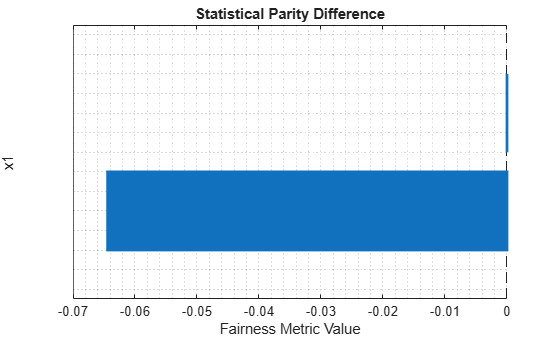For the nonreference group (`Male`), the SPD value is the difference between the probability of a patient being in the positive class (`Nonsmoker`) when the sensitive attribute value is `Male` and the probability of a patient being in the positive class when the sensitive attribute value is `Female` (in the reference group). Ideally, SPD values are close to 0.

To try to improve the nonreference group SPD value, you can adjust the model predictions by using the `fairnessThresholder` function. The function searches for an optimal score threshold to maximize accuracy while satisfying fairness bounds. For observations in the critical region below the optimal threshold, the function changes the labels so that the fairness constraints hold for the reference and nonreference groups. By default, when you use the SPD bias metric, the function tries to find a score threshold such that the SPD value for the nonreference group is in the range [–0.05,0.05].

```fairnessMdl = fairnessThresholder(mdl,validationX, ... validationAttribute,validationY, ... BiasMetric="StatisticalParityDifference")```
```fairnessMdl = fairnessThresholder with properties: Learner: [1x1 classreg.learning.classif.CompactClassificationSVM] SensitiveAttribute: [50x1 categorical] ReferenceGroups: Female ResponseName: 'Y' PositiveClass: Nonsmoker ScoreThreshold: 0.5116 BiasMetric: 'StatisticalParityDifference' BiasMetricValue: -0.0209 BiasMetricRange: [-0.0500 0.0500] ValidationLoss: 0.1200 ```

`fairnessMdl` is a `fairnessThresholder` model object.

Note that the updated nonreference group SPD value is closer to 0.

`newNonReferenceSPD = fairnessMdl.BiasMetricValue`
```newNonReferenceSPD = -0.0209 ```

Use the new score threshold to adjust the validation data predictions. The `predict` function of the `fairnessMdl` object adjusts the prediction of each observation whose maximum score is less than the score threshold. If the observation is in the nonreference group, the function predicts the observation into the positive class. If the observation is in the reference group, the function predicts the observation into the negative class. These adjustments do not always result in a change in the predicted label.

```fairnessLabels = predict(fairnessMdl,validationX, ... validationAttribute);```

Find the observations whose predictions are switched by `fairnessMdl`.

```differentIdx = (labels ~= fairnessLabels); differentX = validationX(differentIdx,:); names(5) = "Switched Prediction";```

Plot the validation data. The color of each point indicates the sensitive attribute group and class label for that observation. Points in squares indicate observations whose labels are switched by the `fairnessThresholder` model.

```figure hold on gscatter(validationX(:,1),validationX(:,2), ... validationAttribute.*validationY) plot(differentX(:,1),differentX(:,2), ... "ks",MarkerSize=8) legend(names) xlabel("Diastolic") ylabel("Systolic") title("Validation Data") hold off```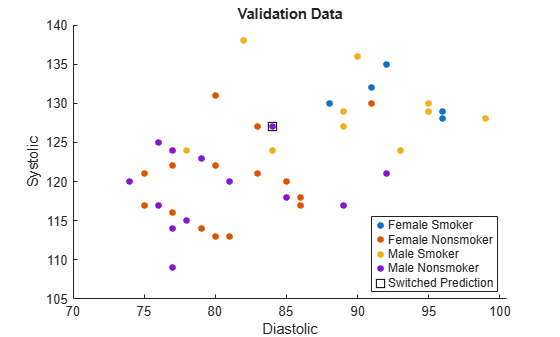The `fairnessThresholder` function uses a technique designed for binary sensitive attributes that contain a reference group and a nonreference group. This example shows how to use the function when the sensitive attribute contains more than two groups.

Read the sample file `CreditRating_Historical.dat` into a table. The predictor data contains financial ratios for a list of corporate customers. The response variable contains credit ratings assigned by a rating agency. Consider the industry sector information as a sensitive attribute.

`creditrating = readtable("CreditRating_Historical.dat");`

Because each value in the `ID` variable is a unique customer ID—that is, `length(unique(creditrating.ID))` is equal to the number of observations in `creditrating`—the `ID` variable is a poor predictor. Remove the `ID` variable from the table, and convert the `Industry` variable to a `categorical` variable.

```creditrating.ID = []; creditrating.Industry = categorical(creditrating.Industry);```

In the `Rating` response variable, combine the `AAA`, `AA`, `A`, and `BBB` ratings into a category of "good" ratings, and the `BB`, `B`, and `CCC` ratings into a category of "poor" ratings.

```Rating = categorical(creditrating.Rating); Rating = mergecats(Rating,["AAA","AA","A","BBB"],"good"); Rating = mergecats(Rating,["BB","B","CCC"],"poor"); creditrating.Rating = Rating;```

Partition the data into a training set, validation set, and test set. Use approximately one third of the observations to create each set.

```rng("default") cv1 = cvpartition(creditrating.Rating,"Holdout",1/3); tblNotForTest = creditrating(training(cv1),:); tblTest = creditrating(test(cv1),:); cv2 = cvpartition(tblNotForTest.Rating,"Holdout",1/2); tblTrain = tblNotForTest(training(cv2),:); tblValidation = tblNotForTest(test(cv2),:);```

In this example, consider industries with high ratios of good to poor ratings as reference groups in the `Industry` sensitive attribute. Compute the ratios using the training data set `tblTrain` and the `grpstats` function.

```info = grpstats(tblTrain,["Industry","Rating"]); goodInfo = info(info.Rating == "good",1:3); poorInfo = info(info.Rating == "poor",1:3); goodToPoorRatio = goodInfo.GroupCount./poorInfo.GroupCount```
```goodToPoorRatio = 12×1 2.0000 1.5122 2.1212 1.3061 1.7778 2.5152 2.4118 1.9394 1.4186 1.1875 ⋮ ```

Define the well-rated industries as those with `goodToPoorRatio` values greater than 2.5. Consider the industry with the highest `goodToPoorRatio` value as the best-rated industry.

`wellRatedIndustries = goodInfo.Industry(goodToPoorRatio > 2.5,:)`
```wellRatedIndustries = 2x1 categorical 6 11 ```
```maximumRatio = max(goodToPoorRatio); bestRatedIndustry = goodInfo.Industry(goodToPoorRatio == maximumRatio,:)```
```bestRatedIndustry = categorical 11 ```

Compute fairness metrics with respect to the sensitive attribute by using the training data. In particular, find the statistical parity difference (SPD) for each group in `Industy`. Specify a good rating as the positive class, and specify the best-rated industry (`11`) as the reference group. Use the `report` and `plot` object functions of `fairnessMetrics` to display the results.

```dataEvaluator = fairnessMetrics(tblTrain,"Rating", ... SensitiveAttributeNames="Industry", ... PositiveClass="good",ReferenceGroup=bestRatedIndustry); report(dataEvaluator,BiasMetrics="StatisticalParityDifference")```
```ans=12×3 table SensitiveAttributeNames Groups StatisticalParityDifference _______________________ ______ ___________________________ Industry 1 -0.075908 Industry 2 -0.14063 Industry 3 -0.062963 Industry 4 -0.1762 Industry 5 -0.10257 Industry 6 -0.027057 Industry 7 -0.035678 Industry 8 -0.08278 Industry 9 -0.15604 Industry 10 -0.19972 Industry 11 0 Industry 12 -0.058364 ```
`plot(dataEvaluator,"StatisticalParityDifference")`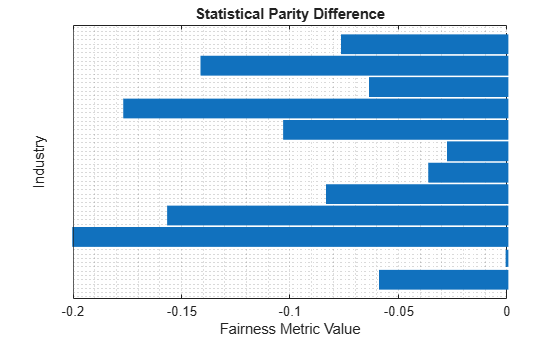For each group g in the sensitive attribute, the SPD value is the difference between the probability of being in the positive class (`good`) when the sensitive attribute value is g and the probability of being in the positive class when the sensitive attribute value is the reference group value (`11`). Ideally, SPD values are close to 0.

Visualize the distribution of SPD values by using a box plot.

```boxchart(dataEvaluator.BiasMetrics.StatisticalParityDifference) ylabel("Statistical Parity Difference") legend("Training Data")```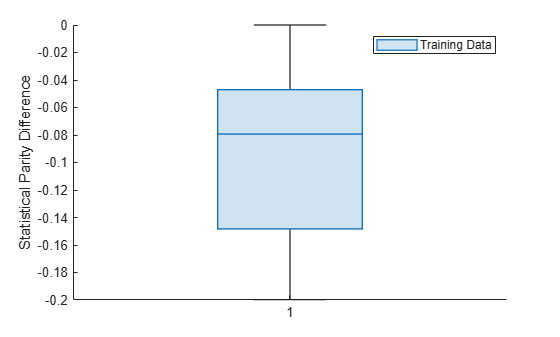The median SPD value is around –0.08.

Train a binary tree classifier using the training data set. Use the trained model to predict labels and compute the classification error on the test data set.

```predictorNames = ["WC_TA","RE_TA","EBIT_TA","MVE_BVTD","S_TA"]; treeMdl = fitctree(tblTrain,"Rating", ... PredictorNames=predictorNames); treePredictions = predict(treeMdl,tblTest); L = loss(treeMdl,tblTest)```
```L = 0.1107 ```

You can adjust model predictions by using the `fairnessThresholder` function. The function uses the validation data to search for an optimal score threshold that maximizes accuracy while satisfying fairness bounds. Use the `ReferenceGroups` name-value argument to specify the well-rated industries (`6` and `11`) as the reference group. All other industries form the nonreference group. Specify the bias metric as the statistical parity difference and the bias metric range as [–0.005,0.005]. Note that these bounds apply to the SPD value for the collective nonreference group, not individual industries in the sensitive attribute.

```fairnessMdl = fairnessThresholder(treeMdl,tblValidation, ... "Industry","Rating", ... PositiveClass="good",ReferenceGroups=wellRatedIndustries, ... BiasMetric="StatisticalParityDifference", ... BiasMetricRange=[-0.005 0.005])```
```fairnessMdl = fairnessThresholder with properties: Learner: [1x1 classreg.learning.classif.CompactClassificationTree] SensitiveAttribute: 'Industry' ReferenceGroups: [2x1 categorical] ResponseName: 'Rating' PositiveClass: 'good' ScoreThreshold: 0.5444 BiasMetric: 'StatisticalParityDifference' BiasMetricValue: 0.0034 BiasMetricRange: [-0.0050 0.0050] ValidationLoss: 0.1198 ```

`fairnessMdl` is a `fairnessThresholder` model object.

Adjust the test set predictions by using the new score threshold, and calculate the classification error.

```newPredictions = predict(fairnessMdl,tblTest); newL = loss(fairnessMdl,tblTest)```
```newL = 0.1183 ```

The new classification error is similar to the original classification error.

Compare the SPD values across the two sets of test predictions: the original predictions computed using `treeMdl` and the adjusted predictions computed using `fairnessMdl`. Specify a good rating as the positive class, and specify the best-rated industry (`11`) as the reference group. Use the `report` and `plot` object functions of `fairnessMetrics` to display the results.

```predEvaluator = fairnessMetrics(tblTest,"Rating", ... SensitiveAttributeNames="Industry", ... Predictions=[treePredictions,newPredictions], ... PositiveClass="good", ... ModelNames=["Original Model","Adjusted Model"], ... ReferenceGroup=bestRatedIndustry); report(predEvaluator,BiasMetric="DisparateImpact")```
```ans=12×5 table Metrics SensitiveAttributeNames Groups Original Model Adjusted Model _______________ _______________________ ______ ______________ ______________ DisparateImpact Industry 1 0.96499 0.95014 DisparateImpact Industry 2 1.0755 1.0634 DisparateImpact Industry 3 0.94643 0.94643 DisparateImpact Industry 4 1.0541 1.0392 DisparateImpact Industry 5 1.0262 1.0132 DisparateImpact Industry 6 1.0186 1.0186 DisparateImpact Industry 7 0.99692 0.96067 DisparateImpact Industry 8 1.077 1.077 DisparateImpact Industry 9 1.0392 1.0103 DisparateImpact Industry 10 1.0781 1.0635 DisparateImpact Industry 11 1 1 DisparateImpact Industry 12 1.0392 1.0225 ```
`plot(predEvaluator,"spd")`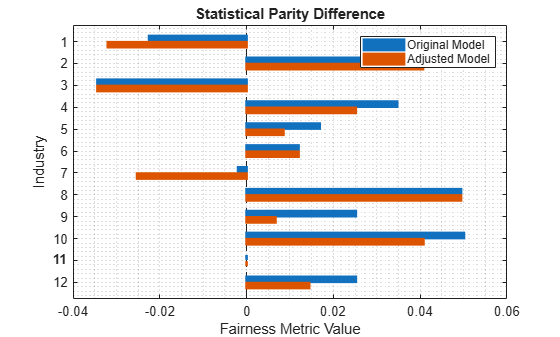Visualize the two distributions of SPD values by using box plots.

```boxchart(predEvaluator.BiasMetrics.StatisticalParityDifference, ... GroupByColor=predEvaluator.BiasMetrics.ModelNames) ylabel("Statistical Parity Difference") legend```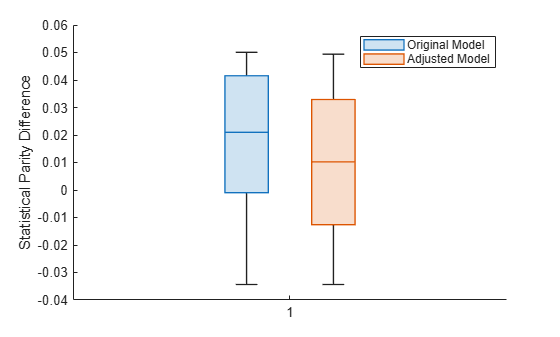The SPD values for the original test set predictions are close to 0, with a median value of approximately 0.02. The SPD values for the adjusted test set predictions have a median value that is slightly closer to 0.

Train a logistic regression model using the `fitglm` function. To adjust the score threshold for classifying observations, pass the model as an input to `fairnessThresholder` using a function handle.

Load the `patients` data set, which contains medical information for 100 patients. Convert the `Gender` and `Smoker` variables to categorical variables. Specify the descriptive category names `Smoker` and `Nonsmoker` rather than `1` and `0`.

```load patients Gender = categorical(Gender); Smoker = categorical(Smoker,logical([1 0]), ... ["Smoker","Nonsmoker"]);```

Create a table containing the continuous predictors `Diastolic` and `Systolic`, the sensitive attribute `Gender`, and the response variable `Smoker`.

`Tbl = table(Diastolic,Systolic,Gender,Smoker);`

Partition the data into training and validation sets. Use half of the observations for training and half of the observations for validation.

```rng("default") % For reproducibility cv = cvpartition(Tbl.Smoker,"Holdout",0.5); trainTbl = Tbl(training(cv),:); validationTbl = Tbl(test(cv),:);```

Train a logistic regression model using the training data `trainTbl` and the `fitglm` function.

```modelspec = "Smoker ~ Diastolic + Systolic"; glmMdl = fitglm(trainTbl,modelspec,Distribution="binomial")```
```glmMdl = Generalized linear regression model: logit(P(Smoker='Nonsmoker')) ~ 1 + Diastolic + Systolic Distribution = Binomial Estimated Coefficients: Estimate SE tStat pValue ________ _______ _______ _________ (Intercept) 116.98 44.939 2.6032 0.0092356 Diastolic -0.54261 0.21577 -2.5147 0.011913 Systolic -0.57999 0.28697 -2.0211 0.043268 50 observations, 47 error degrees of freedom Dispersion: 1 Chi^2-statistic vs. constant model: 54, p-value = 1.89e-12 ```

As indicated in the linear regression model equation, `Nonsmoker` is the positive class. That is, an observation with a predicted score greater than 0.5 is predicted to be a nonsmoker.

Create a function handle to the `predict` function of the `GeneralizedLinearModel` object `glmMdl`.

`f = @(T) predict(glmMdl,T);`

Create a `fairnessThresholder` object by using the function handle `f` and the validation data `validationTbl`. The function searches for an optimal score threshold to maximize accuracy while satisfying fairness bounds. Specify the bias metric range so that the disparate impact value for the nonreference group is in the range [0.9,1.1].

When you pass a classification model as a function handle, you must specify the positive class.

```fairnessMdl = fairnessThresholder(f,validationTbl, ... "Gender","Smoker", ... BiasMetricRange=[0.9 1.1], ... PositiveClass=categorical("Nonsmoker"))```
```fairnessMdl = fairnessThresholder with properties: Learner: @(T)predict(glmMdl,T) SensitiveAttribute: 'Gender' ReferenceGroups: Female ResponseName: 'Smoker' PositiveClass: Nonsmoker ScoreThreshold: 0.8087 BiasMetric: 'DisparateImpact' BiasMetricValue: 0.9538 BiasMetricRange: [0.9000 1.1000] ValidationLoss: 0.1600 ```
`omega = fairnessMdl.ScoreThreshold`
```omega = 0.8087 ```

`fairnessMdl` is a `fairnessThresholder` model object. For each observation with a score in the range (1–`omega`,`omega`), the `predict` function of the `fairnessMdl` object adjusts the prediction. If the observation is in the nonreference group (`Male`), the function predicts the observation into the positive class (`Nonsmoker`). If the observation is in the reference group (`Female`), the function predicts the observation into the negative class (`Smoker`).

Adjust the predictions for the entire data set `Tbl` by using the new score threshold.

`fairnessLabels = predict(fairnessMdl,Tbl)`
```fairnessLabels = 100x1 categorical Smoker Nonsmoker Smoker Nonsmoker Nonsmoker Nonsmoker Smoker Nonsmoker Nonsmoker Nonsmoker Nonsmoker Nonsmoker Nonsmoker Smoker Nonsmoker Smoker Smoker Nonsmoker Nonsmoker Nonsmoker Nonsmoker Nonsmoker Nonsmoker Smoker Smoker Nonsmoker Nonsmoker Nonsmoker Nonsmoker Smoker ⋮ ```

## Algorithms

expand all

 Kamiran, Faisal, Asim Karim, and Xiangliang Zhang. "Decision Theory for Discrimination-Aware Classification." 2012 IEEE 12th International Conference on Data Mining: 924-929.